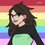# A question about the omega functions $\Omega (n)$ and $\omega (n)$

Given a random positive number $n$, is there a way to determine the number of prime factors of $n$ without factorizing $n$?

There are several useful primality tests for a given number, such as the Miller-Rabin primality test, but is there a test to determine whether number is a semiprime or a 3-almost prime, etc.? Put another way, how can one find either the total number of prime factors (counted with multiplicity) or the number of distinct prime factors without factoring $n$?

After some digging in Wikipedia, I found that these two properties, the number of prime factors to muliplicity and number of distinct prime factors, could be described, respectively, by the functions $\Omega (n)$ and $\omega (n)$.

Thus, we have another way to phrase the question: "Is there a way to calculate either of the omega functions for $n$ without knowing any of the factors of $n$?"

There wasn't much information on those functions at Wikipedia though, and I couldn't parse the information in the notes for the related OEIS sequences.

Here are some links that may be relevant: Prime factor; Prime power decomposition; A001222, The number of prime factors counted with multiplicity, $\Omega (n)$ ; A001221, The number of distinct primes dividing n, $\omega (n)$6 years, 11 months ago

This discussion board is a place to discuss our Daily Challenges and the math and science related to those challenges. Explanations are more than just a solution — they should explain the steps and thinking strategies that you used to obtain the solution. Comments should further the discussion of math and science.

When posting on Brilliant:

• Use the emojis to react to an explanation, whether you're congratulating a job well done , or just really confused .
• Ask specific questions about the challenge or the steps in somebody's explanation. Well-posed questions can add a lot to the discussion, but posting "I don't understand!" doesn't help anyone.
• Try to contribute something new to the discussion, whether it is an extension, generalization or other idea related to the challenge.

MarkdownAppears as
*italics* or _italics_ italics
**bold** or __bold__ bold
- bulleted- list
• bulleted
• list
1. numbered2. list
1. numbered
2. list
Note: you must add a full line of space before and after lists for them to show up correctly
paragraph 1paragraph 2

paragraph 1

paragraph 2

[example link](https://brilliant.org)example link
> This is a quote
This is a quote
    # I indented these lines
# 4 spaces, and now they show
# up as a code block.

print "hello world"
# I indented these lines
# 4 spaces, and now they show
# up as a code block.

print "hello world"
MathAppears as
Remember to wrap math in $$ ... $$ or $ ... $ to ensure proper formatting.
2 \times 3 $2 \times 3$
2^{34} $2^{34}$
a_{i-1} $a_{i-1}$
\frac{2}{3} $\frac{2}{3}$
\sqrt{2} $\sqrt{2}$
\sum_{i=1}^3 $\sum_{i=1}^3$
\sin \theta $\sin \theta$
\boxed{123} $\boxed{123}$